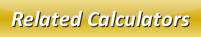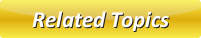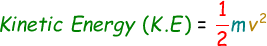Potential Energy CalculatorKinetic Energy CalculatorMechanical Energy CalculatorConservation of EnergyEnergyRenewable Sources of Energy

# Kinetic Energy Calculator

Kinetic Energy can be explained as the energy of motion. When an object is at motion with some velocity and mass is known as kinetic energy. Therefore, all the objects around you moving possess kinetic energy.

The kinetic energy can be calculate with the help of this below formula:where,
K.E = Kinetic Energy [Joules]
m = Mass of the object [Kilograms]
v = Velocity [m/s]

With the help of our online kinetic energy calculator you will be able to calculate the kinetic energy of a moving object instantly. Enter the mass and velocity in the below calculator and click calculate button to get the result.

 Mass of the object (m): [Kilograms] Velocity (v): [m/s] Kinetic Energy (K.E): [Joules]

Latest Calculator Release

Average Acceleration Calculator

Average acceleration is the object's change in speed for a specific given time period. ...

Free Fall Calculator

When an object falls into the ground due to planet's own gravitational force is known a...

Torque Calculator

Torque is nothing but a rotational force. In other words, the amount of force applied t...

Average Force Calculator

Average force can be explained as the amount of force exerted by the body moving at giv...

Angular Displacement Calculator

Angular displacement is the angle at which an object moves on a circular path. It is de...